2021 NFL Comeback Player of the Year Odds
+175
1.75 to 1Dak Prescott
21.9% implied probability

+550
5.5 to 1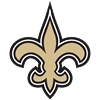Jameis Winston
9.3% implied probability

+650
6.5 to 1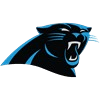Christian McCaffrey
8.0% implied probability

+650
6.5 to 1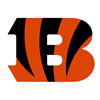Joe Burrow
8.0% implied probability

+1100
11 to 1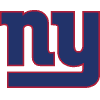Saquon Barkley
5.0% implied probability

+1400
14 to 1Sam Darnold
4.0% implied probability

+1400
14 to 1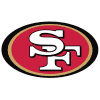George Kittle
4.0% implied probability

No changes have been recorded yet.
+1400
14 to 1Jimmy Garoppolo
4.0% implied probability

+1600
16 to 1Nick Bosa
3.5% implied probability

+1600
16 to 1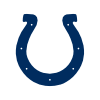Carson Wentz
3.5% implied probability

+1800
18 to 1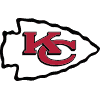Laurent Duvernay-Tardif
3.2% implied probability

No changes have been recorded yet.
+2000
20 to 1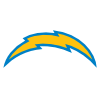Derwin James
2.9% implied probability

+2000
20 to 1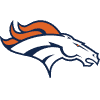Courtland Sutton
2.9% implied probability

No changes have been recorded yet.
+2000
20 to 1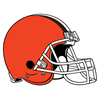Odell Beckham
2.9% implied probability

+2500
25 to 1Von Miller
2.3% implied probability

+2500
25 to 1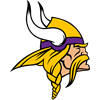Danielle Hunter
2.3% implied probability

+3500
35 to 1James Conner
1.7% implied probability

No changes have been recorded yet.
+3500
35 to 1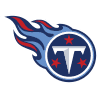Julio Jones
1.7% implied probability

+3500
35 to 1Anthony Barr
1.7% implied probability

No changes have been recorded yet.
+3500
35 to 1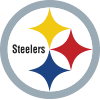Melvin Ingram
1.7% implied probability

No changes have been recorded yet.
+3500
35 to 1Devin Bush
1.7% implied probability

No changes have been recorded yet.
+4000
40 to 1Leighton Vander Esch
1.5% implied probability

No changes have been recorded yet.
+5000
50 to 1O.J. Howard
1.2% implied probability

No changes have been recorded yet.
+5000
50 to 1Richard Sherman
1.2% implied probability

No changes have been recorded yet.